Next: What is the vertex Up: Convex Polyhedron Previous: What is convex hull?   Contents

What is the Minkowski-Weyl theorem for convex polyhedra?

The Minkowski-Weyl Theorem states every polyhedron is finitely generated and every finitely generated set is a polyhedron. More precisely, for two subsets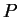andof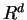,denotes the Minkowski sum ofand: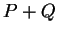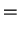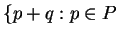and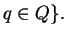Theorem 5 (Minkowski-Weyl's Theorem)   For a subsetof, the following statements are equivalent:
(a)
P is a polyhedron, i.e., for some real (finite) matrix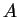and real vector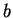,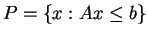;

(b)
There are finite real vectors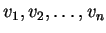and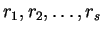insuch that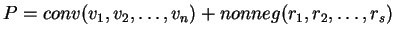.

Thus, every polyhedron has two representations of type (a) and (b), known as (halfspace) H-representation and (vertex) V-representation, respectively. A polyhedron given by H-representation (V-representation) is called H-polyhedron (V-polyhedron).Next: What is the vertex Up: Convex Polyhedron Previous: What is convex hull?   Contents
Komei Fukuda 2004-08-26